### Home > MC1 > Chapter 4 > Lesson 4.2.3 > Problem4-72

4-72.
1. . The following number lines are missing some numbers. Sketch each one on your paper and use the information provided to complete the number lines. Homework Help ✎

1.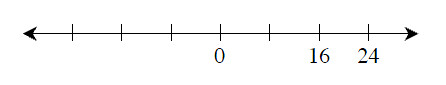2.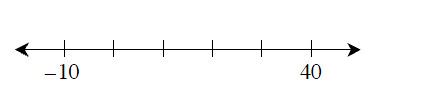3.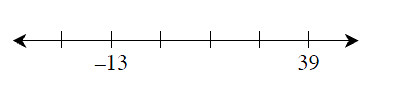4.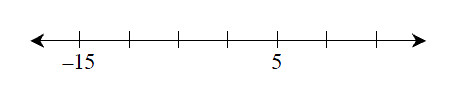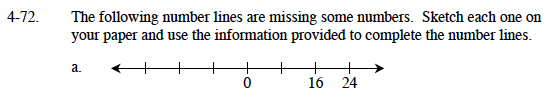Determine which number each tick mark represents. Refer to problem MC1 4-62 for help.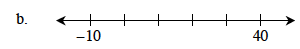Determine which number each tick mark represents.

The distance from 40 to −10 is 50. (Remember, you have to get from 40 to 0, which is 40 units, and from 0 to −10, which is 10 more units.)

50 units divided into 5 sections can be represented as:

$\frac{50}{5}\;=\;{10}$

Each tick mark represents 10 units.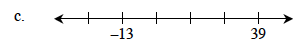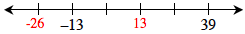Some of the answers have been filled in for you. Remember to fill in the rest of them!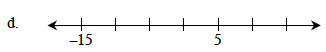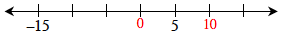Some of the answers have been filled in for you. Remember to fill in the rest of them!